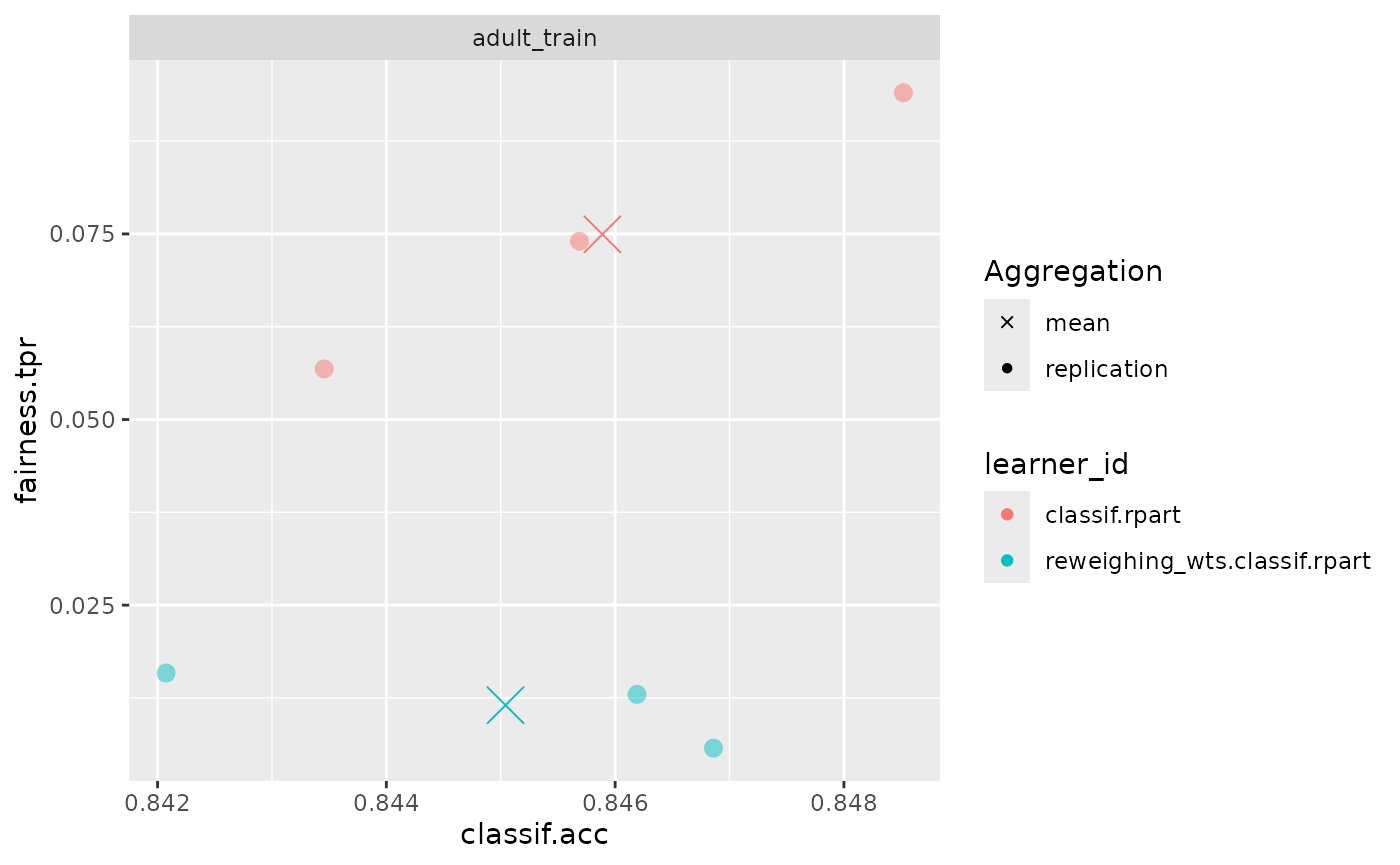## Introduction: Fairness Pipeline Operators

Given we detected some form of bias during bias auditing, we are often interested in obtaining fair(er) models. There are several ways to achieve this, such as collecting additional data or finding and fixing errors in the data, but given there are no biases in the labelling process one other option is to debias models using either preprocessing, postprocessing and inprocessing methods. mlr3fairness provides some operators as PipeOps for mlr3pipelines. If you are not familiar with mlr3pipelines, the mlr3 book

We again showcase debiasing using the adult_train task:

library(mlr3fairness)
library(mlr3pipelines)
library(mlr3)

task = tsk("adult_train")

## Reweighing algorithms

mlr3fairness implements 2 reweighing-based algorithms: reweighing_wts and reweighing_os. reweighing_wts adds observation weights to a Task that can counteract imbalances between the conditional probabilities $$P(Y | pta)$$.

key output.num input.type.train input.type.predict output.type.train

We fist instantiate the PipeOp:

p1 = po("reweighing_wts")

t1 = p1$train(list(task))[] Often we directly combine the PipeOp with a Learner to automate the preprocessing (see learner_rw). Below we instantiate a small benchmark set.seed(4321) learner = lrn("classif.rpart", cp = 0.005) learner_rw = as_learner(po("reweighing_wts") %>>% learner) grd = benchmark_grid(list(task), list(learner, learner_rw), rsmp("cv", folds=3)) bmr = benchmark(grd) We can now compute the metrics for our benchmark and see if reweighing actually improved fairness, measured via True Positive Rate (TPR) and classification accuracy (ACC): bmr$aggregate(msrs(c("fairness.tpr", "fairness.acc")))
#> 2:            cv     3   0.01151982    0.1054431
fairness_accuracy_tradeoff(bmr, msr("fairness.tpr"))